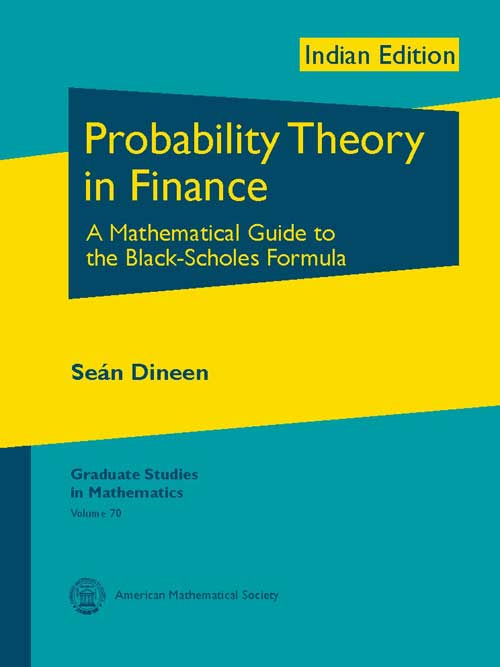Probability Theory in Finance: A Mathematical Guide to the Black-Scholes Formula
Seán Dineen
Price
1325.00
ISBN
9780821868812
Language
English
Pages
312
Format
Paperback
Dimensions
180 x 240 mm
Year of Publishing
2011
Territorial Rights
Restricted
Imprint
American Mathematical Society
Catalogues

The use of the Black-Scholes model and formula is pervasive in financial markets. There are very few undergraduate textbooks available on the subject and, until now, almost none written by mathematicians. Based on a course given by the author, the goal of this book is to introduce advanced undergraduates and beginning graduate students studying the mathematics of finance to the Black-Scholes formula. The author uses a first-principles approach, developing only the minimum background necessary to justify mathematical concepts and placing mathematical developments in context.

The book skillfully draws the reader toward the art of thinking mathematically and then proceeds to lay the foundations in analysis and probability theory underlying modern financial mathematics. It rigorously reveals the mathematical secrets of topics such as abstract measure theory, conditional expectations, martingales, Wiener processes, the Itô calculus, and other ingredients of the Black-Scholes formula. In explaining these topics, the author uses examples drawn from the universe of finance. The book also contains many exercises, some included to clarify simple points of exposition, others to introduce new ideas and techniques, and a few containing relatively deep mathematical results.

With the modest prerequisite of a first course in calculus, the book is suitable for undergraduates and graduate students in mathematics, finance, and economics and can be read, using appropriate selections, at a number of levels.

Seán Dineen, University College Dublin, Ireland.
1. Money and markets
2. Fair games
3. Set theory
4. Measurable functions
5. Probability spaces
6. Expected values
7. Continuity and integrability
8. Conditional expectation
9. Martingales
10. The Black-Scholes formula
11. Stochastic integration
Solutions
Bibliography
Index
THE BOOKPOINT (INDIA) PVT. LTD.